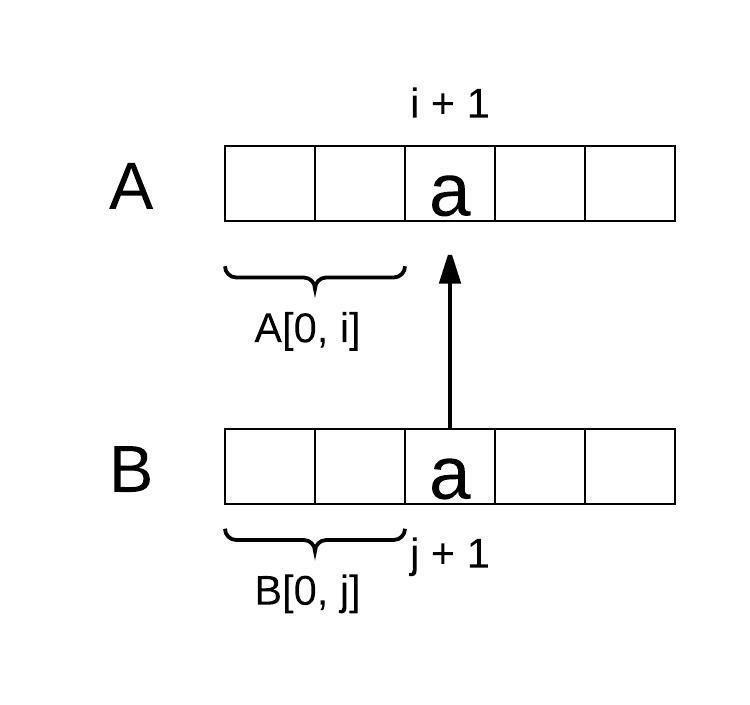Select Page

Dynamic programming can be very challenging in that:

• It is hard to find out whether the question can be solved by dynamic programming or not.
• It is hard to figure out the correct reduction transition function.
• It is hard to implement the algorithm correctly.

Let’s take a look at one typical dynamic programming problem to understand how each step works.

## Wildcard Matching Problem

Implement wildcard pattern matching with support for ‘?’ and ‘*’.

``````'?' Matches any single character.
'*' Matches any sequence of characters (including the empty sequence).

The matching should cover the entire input string (not partial).

The function prototype should be:
bool isMatch(const char *s, const char *p)

Some examples:
isMatch("aa","a") ? false
isMatch("aa","aa") ? true
isMatch("aaa","aa") ? false
isMatch("aa", "*") ? true
isMatch("aa", "a*") ? true
isMatch("ab", "?*") ? true
isMatch("aab", "c*a*b") ? false``````

### Dynamic Programming

We don’t know if this problem can be solved by dynamic program algorithm or not until we can find correct reduction transition.

### Reduction Transition Function

A reduction transition function `reduce the problem from bigger scale to smaller scale`.

To find out the reduction transition function, let’s assume:

• We have string `A` and string `B`
• We know whether `A[0, i]` and its subsequence matches `B[0, j]` and its subsequence.
• Can we determine whether `A[0, i + 1]` matches `B[0, j + 1]`.
• We use `~` to represents matches with.

If we use match[i][j] to represent whether A[0, i] ~ B[0, j], then we are trying to figure out match[i + 1][j + 1]. There are three different cases:

#### B[j] is a regular character

match[i + 1][j + 1] = match[i][j] && A[i + 1] == B[j + 1]

In this case, `match[i + 1][j + 1]` is determined by whether `B[j] ~ A[i]` and whether `A[0, i] ~ B[0, j]`, which is `match[i][j]`. The following chart illustrates this case:Case 1: B[j + 1] is a regular char

#### B[j] is ‘?’

match[i][j] = match[i][j]

Since ‘?’ only matches with any single character, `match[j + 1][j + 1]` is determined by whether match `B[0, j] ~ A[0, i]`, which is `match[i][j]`. The following picture illustrates this case:

#### B[j] is ‘*’

match[i + 1][j + 1] = match[i][j + 1] || match[i + 1][j]

‘*’ can match with any sequence or empty sequence, there are two cases:

• B[j] matches empty string: then `match[i + 1][j + 1] = match[i + 1][j]`.
• B[j] matches with multiple character, which means `B[j + 1]` already been used to match with `A[0, i]`, it is used to match `A[i + 1]` again, then `match[i + 1][j + 1] = match[i][j + 1]`.

The following picture illustrate this case:

### Implementation

We can use a matrix to track `match[i][j]`, the following chart shows how the move on the matrix works:

Consider that we need to look back to `match[i - 1][j - 1]` and `match[i][j - 1]`. If we only allocate `match[len(A)][len(B)]`, when `i = 0` or `j = 0`, we need to handle the transition separately, which makes the code verbose. So instead, we allocate `matches[len(A) + 1][len(b) + 1]`, but we need to initiate the first row in s special way.

The following code implements the algorithm

``````public boolean isMatch(String s, String p) {
int len1 = s.length();
int len2 = p.length();
boolean[][] isMatch = new boolean[len1 + 1][len2 + 1];
isMatch = true;
for (int i = 0; i<len2; i++) {
if (p.charAt(i) == '*') {
isMatch[i + 1] = true;
} else {
break;
}
}

for (int i = 0; i<len1; i++) {
for (int j = 0; j<len2; j++) {
if (p.charAt(j) == '?' || s.charAt(i) == p.charAt(j)) {
isMatch[i + 1][j + 1] = isMatch[i][j];
} else if (p.charAt(j) == '*') {
isMatch[i + 1][j + 1] = isMatch[i][j + 1] || isMatch[i + 1][j];
} else {
isMatch[i + 1][j + 1] = false;
}
}
}
return isMatch[len1][len2];
}``````

### Improvements

Replace the matrix with a rolling array. This can be done easily, we only need to remember a special value, which is `match[i][j]`. The following code implements the algorithm:

``````public boolean isMatch(String s, String p) {
int len1 = s.length();
int len2 = p.length();
boolean[] isMatch = new boolean[len2 + 1];
isMatch = true;
for (int i = 0; i<len2; i++) {
if (p.charAt(i) == '*') {
isMatch[i + 1] = true;
} else {
break;
}
}
boolean oneCharMatch = false;
for (int i = 0; i<len1; i++) {
// update oneCharMatch
oneCharMatch = isMatch;
for (int j = 0; j<len2; j++) {
boolean match = false;
if (p.charAt(j) == '?' || s.charAt(i) == p.charAt(j)) {
match = oneCharMatch;
} else if (p.charAt(j) == '*') {
match = isMatch[j + 1] || isMatch[j];
}
oneCharMatch = isMatch[j + 1];
isMatch[j + 1] = match;
}
// update first char match, after first row should always set false
isMatch = false;
}
return isMatch[len2];
}``````